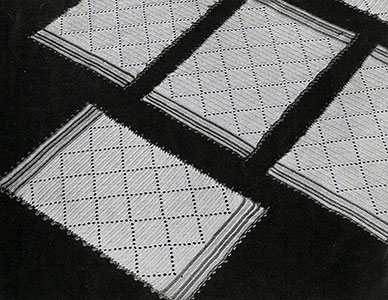## Diamond Luncheon Set Pattern #7006Printer-friendly versionMaterials:
Choose one of the following threads in White or Ecru:
J. & P. Coats Knit-Cro-Sheen, 4 balls of White or Ecru and 1 ball each of 4 contrasting colors to be noted as A - B - C and D.
Clark's O.N.T. Lustersheen, 3 skeins of White or Ecru and 1 skein each of 4 contrasting colors to be noted as A - B - C and D.
Milward's steel crochet hook No. 5.

When completed, center mat and place mats measure each about 9 x 17 inches.

Place Mat. With Ecru, ch 88, turn. 1st row: D c in 4th ch from hook, d c in each of 12 ch (14 d c including turning ch), ch 2, skip 2 ch, d c in each of 26 ch, ch 2, skip 2 ch, d c in each of 26 ch, ch 2, d c in each of 14 ch, ch 3, turn. 2nd row: D c in each of 11 d c, * ch 2, 2 d c in sp, ch 2, skip 2 d c, d c in each of 22 d c. Repeat from * across, ending with 12 d c, ch 3, turn. 3rd row: D c in each of 9 d c, * ch 2, 2 d c in sp, d c in each of 2 d c, 2 d c in sp, ch 2, skip 2 d c, d c in each of 18 d c. Repeat from * across, ending with 10 d c, ch 3, turn. 4th row: D c in each of 7 d c, * ch 2, 2 d c in sp, d c in each of 6 d c, 2 d c in sp, ch 2, skip 2 d c, d c in each of 14 d c. Repeat from * across, ending with 8 d c, ch 3, turn. 5th row: D c in each of 5 d c, * ch 2, 2 d c in sp, d c in each of 10 d c, 2 d c in sp, ch 2, skip 2 d c, d c in each of 10 d c. Repeat from * across, ending with 6 d c, ch 3, turn. 6th row: D c in each of 3 d c, * ch 2, 2 d c in sp, d c in each of 14 d c, 2 d c in sp, ch 2, skip 2 d c, d c in each of 6 d c, repeat from * across, ending with 4 d c, ch 3, turn. 7th row: D c in d c, * ch 2, 2 d c in sp, d c in each of 18 d c, 2 d c in sp, ch 2, skip 2 d c, d c in each of 2 d c, repeat from * across, ending with 2 d c, ch 3, turn. 8th row: * 2 d c in sp, d c in each of 22 d c, 2 d c in sp, ch 2, repeat from * across, ending with ch 1, 1 d c, ch 3, turn. 9th row: D c in sp, * ch 2, skip 2 d c, d c in each of 22 d c, ch 2, 2 d c in sp, repeat from * across, ending with 2 d c, ch 3, turn. 10th row: D c in d c, 2 d c in sp, * ch 2, skip 2 d c, d c in each of 18 d c, ch 2, 2 d c in sp, d c in each of 2 d c, 2 d c in sp, repeat from * across, ending with 4 d c, ch 3, turn. 11th row: D c in each of 3 d c, 2 d c in sp, * ch 2, skip 2 d c, d c in each of 14 d c, ch 2, 2 d c in sp, d c in each of 6 d c, 2 d c in sp, repeat from * across, ending with 6 d c, ch 3, turn. 12th row: D c in each of 5 d c, 2 d c in sp, * ch 2, skip 2 d c, d c in each of 10 d c, ch 2, 2 d c in sp, d c in each of 10 d c, 2 d c in sp, repeat from * across, ending with 8 d c, ch 3, turn. 13th row: D c in each of 7 d c, 2 d c in sp, * ch 2, skip 2 d c, d c in each of 6 d c, ch 2, 2 d c in sp, d c in each of 14 d c, 2 d c in sp, repeat from * across, ending with 10 d c, ch 3, turn. 14th row: D c in each of 9 d c, 2 d c in sp, * ch 2, skip 2 d c, d c in each of 2 d c, ch 2, 2 d c in sp, d c in each of 18 d c, 2 d c in sp, repeat from * across, ending with 12 d c. This completes a diamond. Ch 3, and repeat from the beginning 3 more times ending with a row same as 1st row. Break off.

Border. The border is worked across the two short ends in half d c. Join color B and work 1 row of half d c of each color alternating colors as follows, and breaking off thread when changing colors: A – C – D – C – A – D – A – C – D. Make other end the same. Join color A and work 1 row of s c all around making a ch-5 p at every 5th st and finish off. Make 4 more mats.